# Basics of Computational Reinforcement Learning

Intelligent behavior arises from the actions of an individual seeking to maximize its received reward signals in a complex and changing world”. Meanwhile, reinforcement learning is the way to realize AI.

In machine learning, reinforcement learning plays an important role. It stems from the system’s decision-making ability to be improved through interacting with the world and evaluating feedback. This tutorial introduces basic concepts and vocabulary in this field. Additionally, the tutorial shows us recent advances in the theory and practice of reinforcement learning.
To start off, the speaker uses a graph to tell us the importance of knowing natural RLDM (reinforcement learning and decision making) for studying artificial RLDM.Then he follows up with four main sections:

## What is computational RL: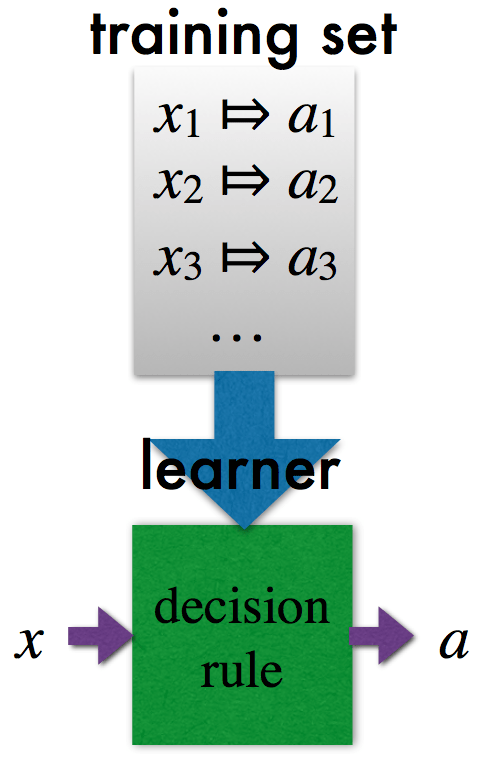The speaker uses an example to explain supervised learning. As we all know, supervised learning is a task that infers a function from labeled training data . That is to say, we should construct a rule that acts like the training set without over-fitting. As shown in the left image, every training set x has the related label a, and we can learn from that. When you input a new dataset, it can show the label belonging to that data .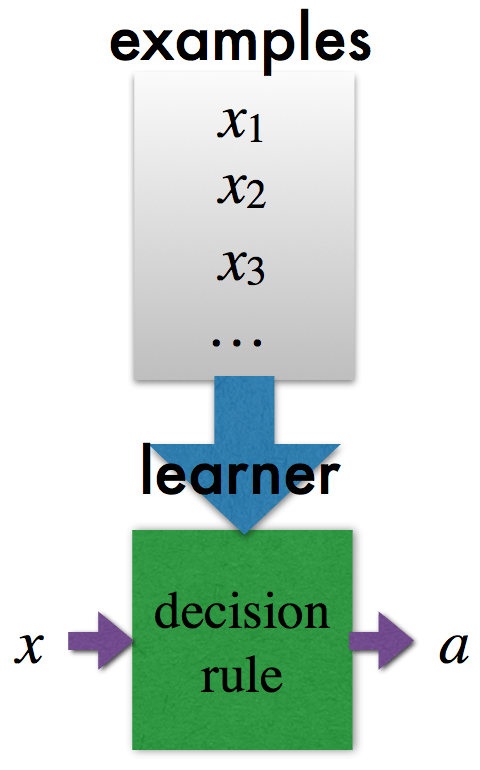Then the speaker introduces unsupervised learning, which is a task that infers a function to describe hidden structure from “unlabeled” data . In this kind of problems, we should cluster similar examples into the same class. But this may not be relevant to what we want. As shown in the left image, we don’t know how to divide the training dataset into different categories, so we let the computer learn the regular pattern, and when you input new data, it can be decided by the learned model.For reinforcement learning, the main difference is the existence of an evaluative feedback value for that choice. But for this problem, the difficulty lies in the fact that we can’t know how good or how bad the feedback is. So what we need to do is to construct a rule to get the maximum expected value. As shown in the left image, for every training dataset, there is not only the related category, but also a feedback value for that choice. After learning the training data, it will find a way to make the prediction that are followed by the feedback values.

The speaker gives three comparisons between sequential and one-shot, sampled and exhaustive, evaluative and supervised and relates different kinds of feedbacks to each other. He then uses the standard to decide which kind the learning functions belong to. For the supervised type, the learner tells the best feedback value. For the exhaustive type, the learner shows every possible training set x. For the one-shot type, there is no relation between the past feedback and the current training set. We can see in the following figure.The reinforcement-learning hypothesis is introduced: “Intelligent behavior arises from the actions of an individual seeking to maximize its received reward signals in a complex and changing world”. Meanwhile, the speaker points out that reinforcement learning is the way to realize AI. There are two problems that we should solve to make it work. Where do reward signals come from? How to develop algorithms that search the space of behaviors to maximize reward signals?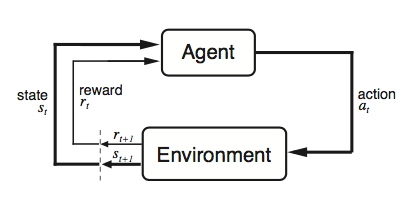Agent-Environment interaction is shown by a diagram. For the agent, there are two problems: How to act to maximize cumulative discounted expected reward? How to make the optimization? For the world function (environment), there are also two problems: How to deliver reward so that agent adopts desirable behavior? How to make this mechanism design? Reward expressiveness is also introduced. It’s used to encourage reaching a goal state. It once contained soft trade-offs, and we should shape rewards to aid learning.

Reward is a kind of expressiveness, it tells people what to do. There are two reward types that are being introduced as follow. The first is to encourage reaching a goal state, and the second is to avoid a failure state. Both these two once used soft trade-offs. Another way to do this is shaping rewards, which can aid learning.

## Planning and learning in Markov Models:

The most popular formalization is Markov decision process (MDP), which provides a mathematical framework for modeling decision making in situations. The process can be described by the following image, it is going to model the environment image as shown above, which has two functions itself: the transition function and the reward function. It undergoes a series of transitions. For the state, it chooses the action, gets some reward, and end up in the new state where it can get a new action and its reward and get the transition to get to another state. If the agent ask the optimal reward, the expected future discounted rewards from some starting s and taking one action a, it will recursively find the optimal reward. We get the functions follow the process.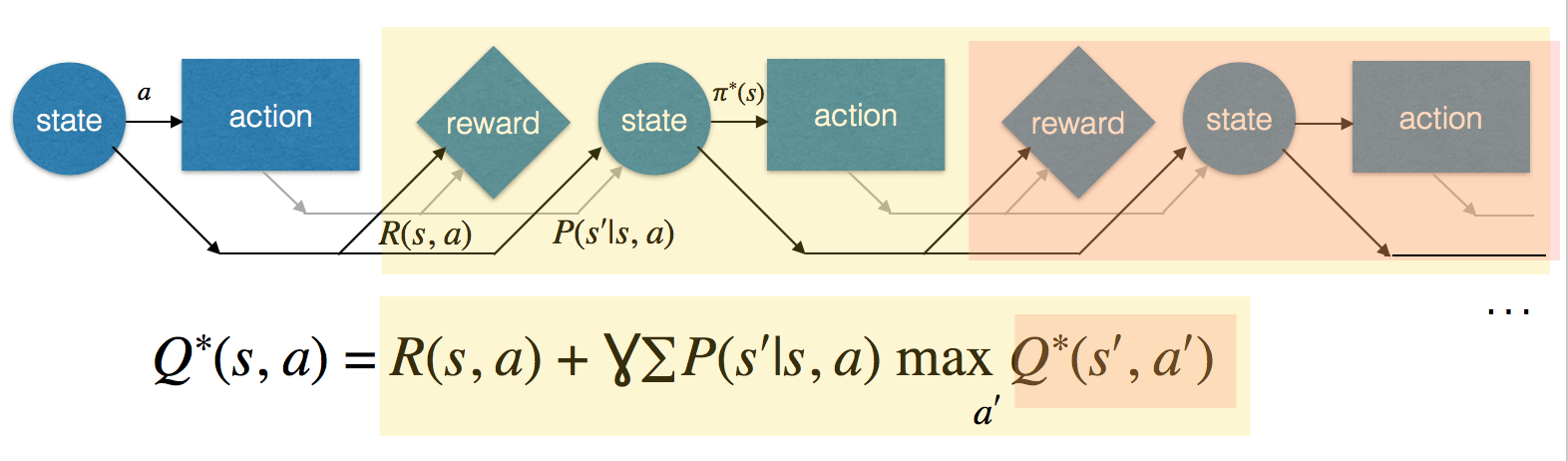Additionally, it uses “find the ball” example to give an explanation of MDP. There are only two actions: rotate left and rotate right. If the dog faces ball, there will be a reward. This model can be used for understanding MDP. The speaker also shows the MDP planning used to solve the Bellman equation:There are mainly three functions: value iteration converges in the limit, which guesses the Q function first and use the iterations to converge to the result; policy iteration converges in finite time, which guesses the Q function first and build the Q function for the policy, then it can converge faster; and linear programming runs in polynomial time, which use the two iterated functions to realize this one.

The above contents didn’t tell about reinforcement learning (RL), it just figures out if someone gives you MDP, what you can do to optimize. So for RL algorithms, the speaker shows two types: model-free learning, which uses experience to directly build Q function, and model-based learning, which builds the transition function and reward function, then compute the Q function. Q-learning is a kind of model-free learning: it first initializes the Q function, we then use the Q function to find or explore the highest action rewards and take it, then observe the transition function and decay the learning rate. This is “off policy” learning. For SARSA, it is similar to Q-learning, but it replaces the update in a different observing transition. This is learning “on policy”.

For model-based learning, it assumes knowledge of transition, the reward function, and the state and action spaces. This defines the model of the world. The speaker uses the simulation lemma to show that long-term value smoothly varies with the MDP parameters, and testify that the model-based approach can get us close to optimal. Additionally, he shows the generalized MDPs.

For the multi-agent setting, two agents may want the same direction, or opposite directions like a competition. The speaker uses a game to illustrate that. And cognitive hierarchy, which was proposed in one-shot normal-form games, has proven the accuracy in modeling human decisions and guiding machine decisions. There are also three subtleties that should be paid attention: how to randomize over sequential behavior? Can player adapt during the interaction? How to handle the enormous strategy space?

## Efficient reinforcement learning:

Here, a game was used to illustrate reinforcement learning. The game is different from tabular reinforcement learning, all you have to do is to play the game and try to learn from that. In this game, the passenger wants to go to the same color as himself. He can choose one of six actions to play and get to that state. So what is the efficiency of reinforcement learning? The convergence is better than not convergence, but “in the limit” guarantees are really weak. We should also consider the convergence rate, sublinear regret and optimal reward. The sample complexity is also considered by the computer scientist.

After that, the speaker shows the definition of Probably Approximately Correct (PAC) MDP, which is giving the pre-condition, including the number of actions, states, and discount factor to the learner, and the learner is to take actions in the states. Every time the learner is in some state, the action taken from that state is nearly optimal. There is a judge for that action, and if it’s worse than the standard, then it is said to have made a mistake. As it is learning, it will inevitably make mistakes, and we can let it make mistakes. But we should mention a bound on the number of mistakes that must hold the prior probability, which will control how many times this will happen. In this process, the speaker points out that we must balance exploration and exploitation. The speaker also points out that model-based RL can be efficient, and different assumptions have different behaviors.

The speaker then shows a brief history of optimism in RL. There are mainly 3 algorithms in history. The speaker focuses on the latest algorithm: R-max algorithm, which can attain near-optimal average reward in polynomial time. According to prior work, in R-MAX, the agent maintains a complete, but possibly inaccurate model of the environment. The agent acts based on optimal policy. The reason why it is called R-MAX is that all actions in all states return the maximum possible reward. It is updated based on the agent’s observations during the execution. R-MAX is simpler and more general than the previous algorithms, it has a built-in mechanism for resolving the exploration vs exploitation dilemma. That is, if it can’t reach unknown states quickly, it’s nearly optimal.

We can see the example of R-max algorithms in visualization and in robotics. In the simulation experiment, one point in the corner wants to go to another place, but there are some roadblocks, so the point of using R-max algorithm is to find a way to realize that. In the robotics experiment, the point is replaced by a robotic dog. From the above examples, we can see that we really need to move from that kind of exhaustive model to sampled model. To do this, the speaker shows three models for learning transitions, and directly jumps to the new learning model: KWIK, which can predict outputs or say “I don’t know” and observe labels. There should be no mistakes, but it can say “I don’t know” m times (there are m inputs). The speaker uses an example to show the mechanism.

Here is how we can use this in reinforcement learning. We can quickly learn the reward transition function in the environment. That’s called the KWIK-Rmax Algorithm. The speaker catalogs several KWIK-learnable classes, and demonstrates their application in experience-efficient reinforcement learning. Another kind of class that we end up doing quick learning is the relocatable action models. This decomposes transitions into state-independent outcomes. Here it uses a robotic example, which is similar to the previous experiment. In this experiment, the robot also changes by doing actions, and there are two types of area, sand and wood. It can only take left, right and forward actions. The states are position and orientation, and the goal is to reach the box as quickly as possible.

The speaker uses another game, a hidden-bit problem to illustrate the learning of unknown structure in DBN model. By keeping statistics on pairwise correlations, it can estimate necessary probabilities and know which ones to trust. The model in the world of objects is try to learn what happens when objects interact. This leads us to very “human like” exploration – objected-oriented MDPs. It provides comparison to show how the algorithms acts in the taxi problem. In the taxi problem, there are three features: the location of the taxi, the location of the passenger, and the passenger’s destination. It is used to explain the dependency of these relationships, and if the learner knows this beforehand, it can learn more quickly. In the following figure, it shows the results of running different algorithms in the taxi problem. Additionally, it is compared with two kinds of people in the last two lines.We can actually learn models very quickly and efficiently that are too big to solve. They are too big to actually do the planning. The speaker uses tree search to discuss this problem. Tree search works forwards from the current state, but it’s not an “efficient state-independent planner” due to branching factor, which is called sparse sampling. Monte-Carlo tree search brings this to a higher level, it’s narrow and deep focused, and repeatedly traverse from the top.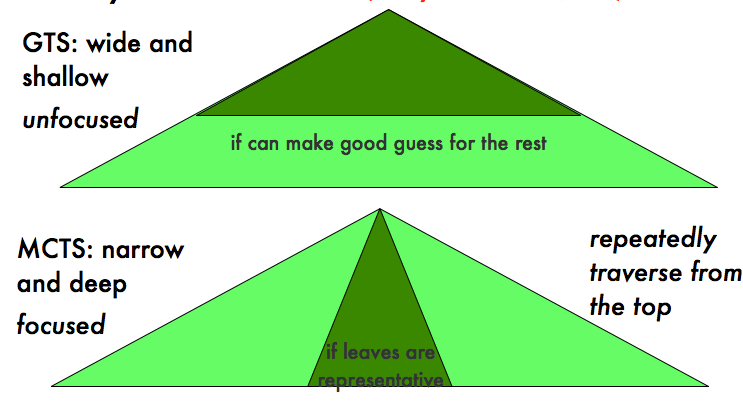The speaker shows an example in the video of learning to walk, the first video is to show how to learn walking against strong winds, and the second video is to show how to walk down the stairs as fast as possible. They all first try to act and find a way to optimize, and then perform iterations to be nearly to optimal. These are to show the optimization approach and reward-rich policy sampling.

## Frontiers:

Here are some empirical methods that the authors concluded, and it shows the learning algorithms results of playing games, compared with people. There are bunches of functions to decide how to determine the rewards, and there are demonstration, human-delivered reward and punishment, natural language and evolution. The speaker also talks about how to maximize the reward to get it optimized in a box eating game, which involves moving around the grid to get to the box, open it, and eat from the box right away. This is to find a behavior from the agent that tends to learn quickly to get high reward. It gives the agent thousands of steps to learn, and find what will maximum the reward it gets. It can initially be given a little bit of shipping reward that will help it be closer to the boxes.

Inverse reinforcement learning also exists. It gives examples of behaviors and get the related rewards. The stochastic policies give us a probability of selecting each action in each state, and it finds reward function that maximizes data likelihood. The speaker also demonstrates a driving game and shows how humans earn rewards. It shows that it’s better to view “rewards” as evidence for an underlying reward function.

The last bit is about the portal principle. Video games can get us to solve very hard puzzles. Our ability to solve challenging problems rests on layering our knowledge.

## Conclusion:

This video gives us a brief description of the fundamental concepts. It uses a lot of classic experiments or ones that were carried out by the speaker to show the process of reinforcement learning. It also gives us the analysis of different algorithms, and emphasizes the importance of rewards in reinforcement learning. We can use the theory that the speaker provides to realize reinforcement learning.

## References:

 Mehryar Mohri, Afshin Rostamizadeh, Ameet Talwalkar (2012) Foundations of Machine Learning, The MIT Press ISBN 9780262018258.
 https://en.wikipedia.org/wiki/Unsupervised_learning
 Nature, 2015
 Sutton & Barton 98
 Brafman, Ronen I., and Moshe Tennenholtz. “R-max-a general polynomial time algorithm for near-optimal reinforcement learning.” Journal of Machine Learning Research 3.Oct (2002): 213-231.

Author: Shixin Gu  | Localized by Synced Global Team: Yuanchao Li